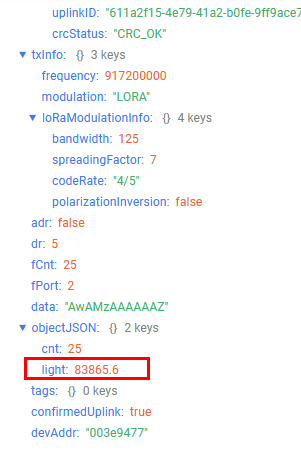# RAK1903 - Sensor Readings at Server not working after certain level

Hi, Has anyone seen an issue where the LoRa readings (at the server) from the RAK1903 stop working after a certain level of LUx is achieved? If I have a serial reading of let us say 136lux then the reading at the server (after decoding) is correct. Although once the serial readings go over 700Lux the readings on the server give an incorrect result. Any clues on this?

Code:
m_lora_app_data.buffer[i++] = 0x02;
m_lora_app_data.buffer[i++] = (uint8_t)(t >> 8);
m_lora_app_data.buffer[i++] = (uint8_t)t;
m_lora_app_data.buffer[i++] = (uint8_t)(h >> 8);
m_lora_app_data.buffer[i++] = (uint8_t)h;
m_lora_app_data.buffer[i++] = (uint8_t)((pre & 0xFF000000) >> 24);
m_lora_app_data.buffer[i++] = (uint8_t)((pre & 0x00FF0000) >> 16);
m_lora_app_data.buffer[i++] = (uint8_t)((pre & 0x0000FF00) >> 8);
m_lora_app_data.buffer[i++] = (uint8_t)(pre & 0x000000FF);
m_lora_app_data.buffer[i++] = (uint8_t)(l >> 8);
m_lora_app_data.buffer[i++] = (uint8_t)l;
m_lora_app_data.buffsize = i;

Decode:
case 0x02: // Weather Sensor data
decoded.temperature = (bytes << 8 | bytes) / 100;
decoded.humidity = (bytes << 8 | + bytes) / 100;
decoded.pressure = (bytes | (bytes << 8) | (bytes << 16) | (bytes << 24)) / 100;
decoded.light = (bytes << 8 | + bytes) / 100;
break;

Tem:21.19C Hum:40.19% Pres:101.36KPa Lig:3626.24lux

humidity:40.19
light:349.44
pressure:101.35
temperature:21.18

Hello Kevin,

I have no problems to get light values of over 700 on my Chirpstack server:

How do you read the light values? How is the variable for the light value `l` defined in your code?

I am using a “pure” light solution, so the encoding and decoding looks slightly different. (ignore the `cnt` as well please)
Decoding on Chirpstack:

``````		case 0x03: // Ambient light sensor data
decoded.light = (bytes | (bytes << 8) | (bytes << 16) | (bytes << 24)) / 10;
decoded.cnt = bytes | (bytes << 8) | (bytes << 16) | (bytes << 24);
break;
``````

Encoding in the application:

1. Setup of the light sensor:
``````		g_opt3001.begin(0x44);

OPT3001_Config newConfig;

newConfig.RangeNumber = B1100;
newConfig.ConvertionTime = B0;
newConfig.Latch = B1;
newConfig.ModeOfConversionOperation = B11;

g_opt3001.writeConfig(newConfig);
``````

Reading data into a uint32_t. I convert the float value into integer by multiplying by 10. and then encoding it into the data packet:

``````		uint32_t light_val_32 = 0;
if (light_result.error == NO_ERROR)
{
light_val_32 = (uint32_t)(light_result.lux * 10);
}

m_lora_app_data.buffsize = 0;
m_lora_app_data.buffer[m_lora_app_data.buffsize++] = 0x03;

m_lora_app_data.buffer[m_lora_app_data.buffsize++] = (uint8_t)((light_val_32 & 0xFF000000) >> 24);
m_lora_app_data.buffer[m_lora_app_data.buffsize++] = (uint8_t)((light_val_32 & 0x00FF0000) >> 16);
m_lora_app_data.buffer[m_lora_app_data.buffsize++] = (uint8_t)((light_val_32 & 0x0000FF00) >> 8);
m_lora_app_data.buffer[m_lora_app_data.buffsize++] = (uint8_t)(light_val_32 & 0x000000FF);

lmh_send(&m_lora_app_data, LMH_UNCONFIRMED_MSG);
``````

Using a 32bit variable I can read even higher values (the OPT3001 can read up to 83000 Lux).
With a LED flashlight on top of the sensor I can get the saturation value of the sensor:Hi Bernd, I am using the Weather_Monitoring.ino (WisBlock/Weather_Monitoring.ino at master · RAKWireless/WisBlock · GitHub) which defines `l` within the code. Looking at your example there is a difference so may I have to try to incorporate this block of code into the Weather_Monitoriing.ino I have. Hopefully is then just a simple update of the decode script with the Chirpstack server. I will let you know how I go. Thanks for the speedy response Kevin

Hi Bernd, Thanks for the help that worked perfectly.

App Code Used:

uint16_t t = temp * 100;
uint16_t h = hum * 100;
uint32_t pre = pres * 100;
uint32_t l = result.lux * 100;
//result: T=28.25C, RH=50.00%, P=958.57hPa, light=100.46 lux
m_lora_app_data.buffer[i++] = 0x02;
m_lora_app_data.buffer[i++] = (uint8_t)(t >> 8);
m_lora_app_data.buffer[i++] = (uint8_t)t;
m_lora_app_data.buffer[i++] = (uint8_t)(h >> 8);
m_lora_app_data.buffer[i++] = (uint8_t)h;
m_lora_app_data.buffer[i++] = (uint8_t)((pre & 0xFF000000) >> 24);
m_lora_app_data.buffer[i++] = (uint8_t)((pre & 0x00FF0000) >> 16);
m_lora_app_data.buffer[i++] = (uint8_t)((pre & 0x0000FF00) >> 8);
m_lora_app_data.buffer[i++] = (uint8_t)(pre & 0x000000FF);
m_lora_app_data.buffer[i++] = (uint8_t)((l & 0xFF000000) >> 24);
m_lora_app_data.buffer[i++] = (uint8_t)((l & 0x00FF0000) >> 16);
m_lora_app_data.buffer[i++] = (uint8_t)((l & 0x0000FF00) >> 8);
m_lora_app_data.buffer[i++] = (uint8_t)(l & 0x000000FF);
m_lora_app_data.buffsize = I;

Chirpstack Decode Used:

case 0x02: // Weather Sensor data
decoded.temperature = (bytes << 8 | bytes) / 100;
decoded.humidity = (bytes << 8 | + bytes) / 100;
decoded.pressure = (bytes | (bytes << 8) | (bytes << 16) | (bytes << 24)) / 100;
decoded.light = (bytes | (bytes << 8) | (bytes << 16) | (bytes << 24)) / 100;
break;We had the examples and solutions tested, but maybe not deep enough.
I always only look into the examples, then start my own code after understanding the principles.